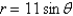# Calculus Early Transcendentals

Mathematics

## Quiz 9 : Parametric Equations and Polar CoordinatesStudy FlashcardsLooking for Calculus Homework Help?

## Quiz 9 :Parametric Equations and Polar Coordinates

Question TypeThe graph of the following curve is given. Find the area that it encloses.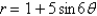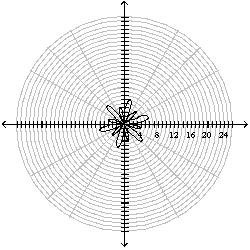Free
Multiple Choice

D

Tags
Choose question tagFind the area of the region that lies inside the first curve and outside the second curve.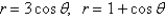Free
Multiple Choice

E

Tags
Choose question tagConsider the polar equation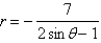.(a) Find the eccentricity and an equation of the directrix of the conic.(b) Identify the conic.(c) Sketch the curve.
Free

(a) eccentricity: 2, directrix: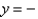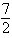(b) hyperbola
(c)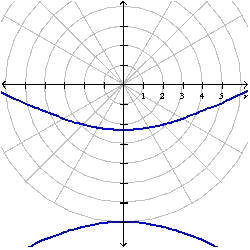Tags
Choose question tagFind the area that the curve encloses.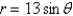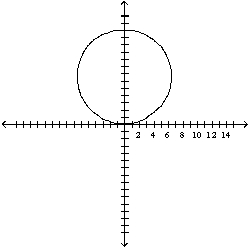Tags
Choose question tagThe orbit of Hale-Bopp comet, discovered in 1995, is an ellipse with eccentricity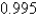and one focus at the Sun. The length of its major axis is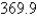AU. [An astronomical unit (AU) is the mean distance between Earth and the Sun, about 93 million miles.] Find the maximum distance from the comet to the Sun. (The perihelion distance from a planet to the Sun is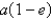and the aphelion distance is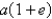.) Find the answer in AU and round to the nearest hundredth.
Multiple Choice
Tags
Choose question tagWrite a polar equation of the conic that has a focus at the origin, eccentricity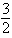, and directrix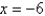. Identify the conic.
Multiple Choice
Tags
Choose question tagThe planet Mercury travels in an elliptical orbit with eccentricity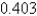. Its minimum distance from the Sun is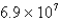km. If the perihelion distance from a planet to the Sun is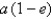and the aphelion distance is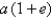, find the maximum distance (in km) from Mercury to the Sun.
Multiple Choice
Tags
Choose question tagFind a polar equation for the curve represented by the given Cartesian equation.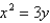Multiple Choice
Tags
Choose question tagWrite a polar equation in r and of an ellipse with the focus at the origin, with the eccentricity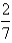and directrix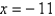.
Multiple Choice
Tags
Choose question tagConsider the polar equation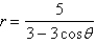.(a) Find the eccentricity and an equation of the directrix of the conic.(b) Identify the conic.(c) Sketch the curve.
Tags
Choose question tagFind the area enclosed by the curve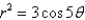.
Tags
Choose question tagFind the length of the polar curve.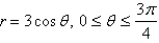Multiple Choice
Tags
Choose question tagWrite a polar equation in r and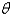of a hyperbola with the focus at the origin, with the eccentricity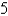and directrix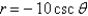.
Multiple Choice
Tags
Choose question tagFind the area of the region enclosed by one loop of the curve.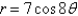Multiple Choice
Tags
Choose question tagFind the Cartesian coordinates of the point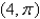.
Multiple Choice
Tags
Choose question tagFind the eccentricity of the conic.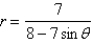Multiple Choice
Tags
Choose question tagWrite a polar equation in r and of an ellipse with the focus at the origin, with the eccentricity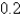and vertex at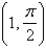.
Multiple Choice
Tags
Choose question tagSketch the polar curve with the given equation.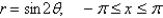Multiple Choice
Tags
Choose question tagFind the polar equation for the curve represented by the given Cartesian equation.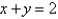Multiple ChoiceFind a Cartesian equation for the curve described by the given polar equation.